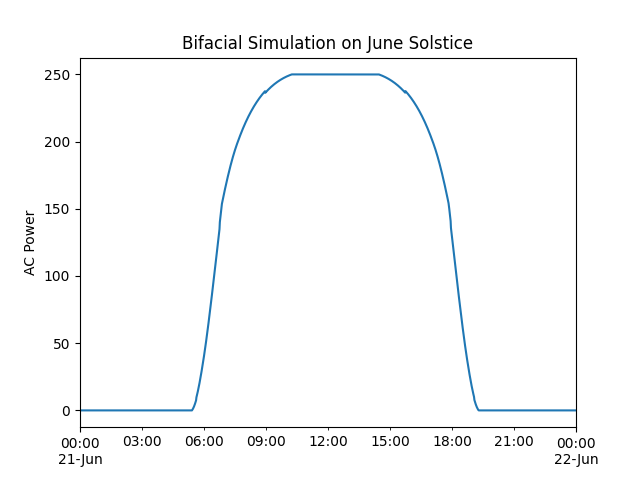# Bifacial Modeling - modelchain#

Example of bifacial modeling using pvfactors and ModelChain

This example shows how to complete a bifacial modeling example using the `pvlib.modelchain.ModelChain` with the `pvlib.bifacial.pvfactors.pvfactors_timeseries()` function to transpose GHI data to both front and rear Plane of Array (POA) irradiance.

Unfortunately `ModelChain` does not yet support bifacial simulation directly so we have to do the bifacial irradiance simulation ourselves. Once the combined front + rear irradiance is known, we can pass that to `ModelChain` and proceed as usual.

Future versions of pvlib may make it easier to do bifacial modeling with `ModelChain`.

```import pandas as pd
from pvlib import pvsystem
from pvlib import location
from pvlib import modelchain
from pvlib.temperature import TEMPERATURE_MODEL_PARAMETERS as PARAMS
from pvlib.bifacial.pvfactors import pvfactors_timeseries
import warnings

# supressing shapely warnings that occur on import of pvfactors
warnings.filterwarnings(action='ignore', module='pvfactors')

# create site location and times characteristics
lat, lon = 36.084, -79.817
tz = 'Etc/GMT+5'
times = pd.date_range('2021-06-21', '2021-6-22', freq='1T', tz=tz)

# create site system characteristics
axis_tilt = 0
axis_azimuth = 180
gcr = 0.35
max_angle = 60
pvrow_height = 3
pvrow_width = 4
albedo = 0.2
bifaciality = 0.75

# load temperature parameters and module/inverter specifications
temp_model_parameters = PARAMS['sapm']['open_rack_glass_glass']
cec_modules = pvsystem.retrieve_sam('CECMod')
cec_module = cec_modules['Trina_Solar_TSM_300DEG5C_07_II_']
cec_inverters = pvsystem.retrieve_sam('cecinverter')
cec_inverter = cec_inverters['ABB__MICRO_0_25_I_OUTD_US_208__208V_']

# create a location for site, and get solar position and clearsky data
site_location = location.Location(lat, lon, tz=tz, name='Greensboro, NC')
solar_position = site_location.get_solarposition(times)
cs = site_location.get_clearsky(times)

# load solar position and tracker orientation for use in pvsystem object
sat_mount = pvsystem.SingleAxisTrackerMount(axis_tilt=axis_tilt,
axis_azimuth=axis_azimuth,
max_angle=max_angle,
backtrack=True,
gcr=gcr)

# created for use in pvfactors timeseries
orientation = sat_mount.get_orientation(solar_position['apparent_zenith'],
solar_position['azimuth'])

# get rear and front side irradiance from pvfactors transposition engine
# explicity simulate on pvarray with 3 rows, with sensor placed in middle row
# users may select different values depending on needs
solar_position['apparent_zenith'],
orientation['surface_azimuth'],
orientation['surface_tilt'],
axis_azimuth,
times,
cs['dni'],
cs['dhi'],
gcr,
pvrow_height,
pvrow_width,
albedo,
n_pvrows=3,
index_observed_pvrow=1
)

# turn into pandas DataFrame

# create bifacial effective irradiance using aoi-corrected timeseries values
)
```

With effective irradiance, we can pass data to ModelChain for bifacial simulation.

```# dc arrays
array = pvsystem.Array(mount=sat_mount,
module_parameters=cec_module,
temperature_model_parameters=temp_model_parameters)

# create system object
system = pvsystem.PVSystem(arrays=[array],
inverter_parameters=cec_inverter)

# ModelChain requires the parameter aoi_loss to have a value. pvfactors
# applies surface reflection models in the calculation of front and back
# irradiance, so assign aoi_model='no_loss' to avoid double counting
# reflections.
mc_bifi = modelchain.ModelChain(system, site_location, aoi_model='no_loss')```<AxesSubplot:title={'center':'Bifacial Simulation on June Solstice'}, ylabel='AC Power'>